maths > wholenumbers

Division: First Principles

what you'll learn...

overview

This page introduces division of whole numbers as -- one number, dividend, is split into the number of parts given by the second number, divisor. The count or measure of one part is the result, quotient. And the remaining count of dividend, that could not be split, is the remainder of the division.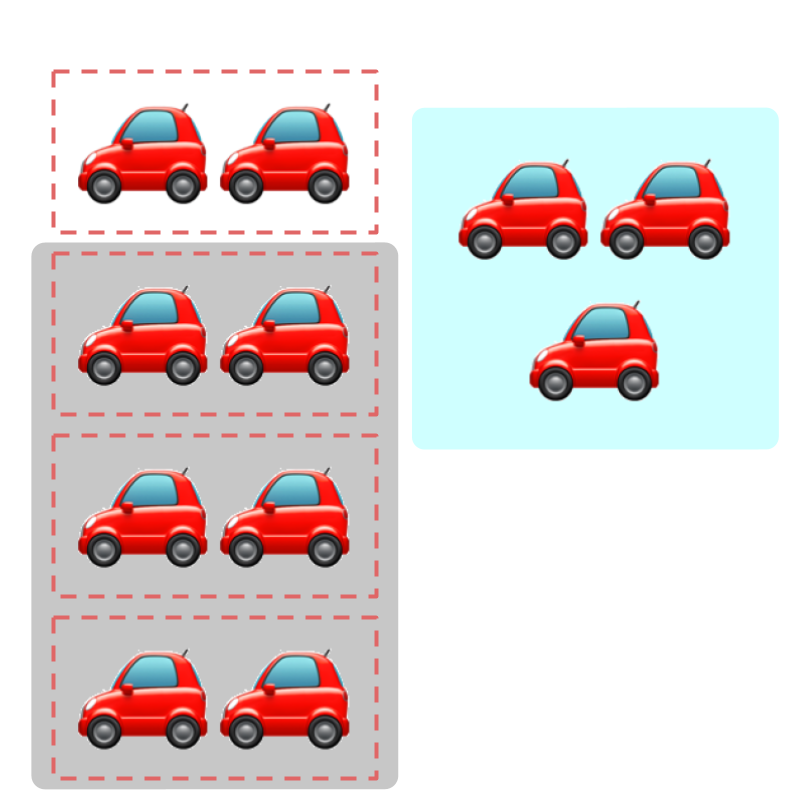Count of 11$11$ is split into 4$4$ parts. The resulting count of one part is quotient 2$2$ and the part that is not split is the remainder 3$3$.

The same is abstracted for division in number-line.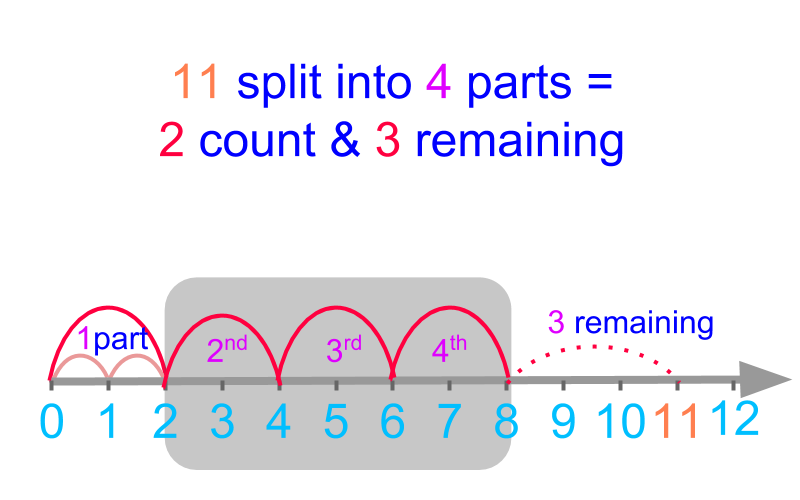The definition of division in first principles form the basis to understanding simplified procedure for division of large numbers.

split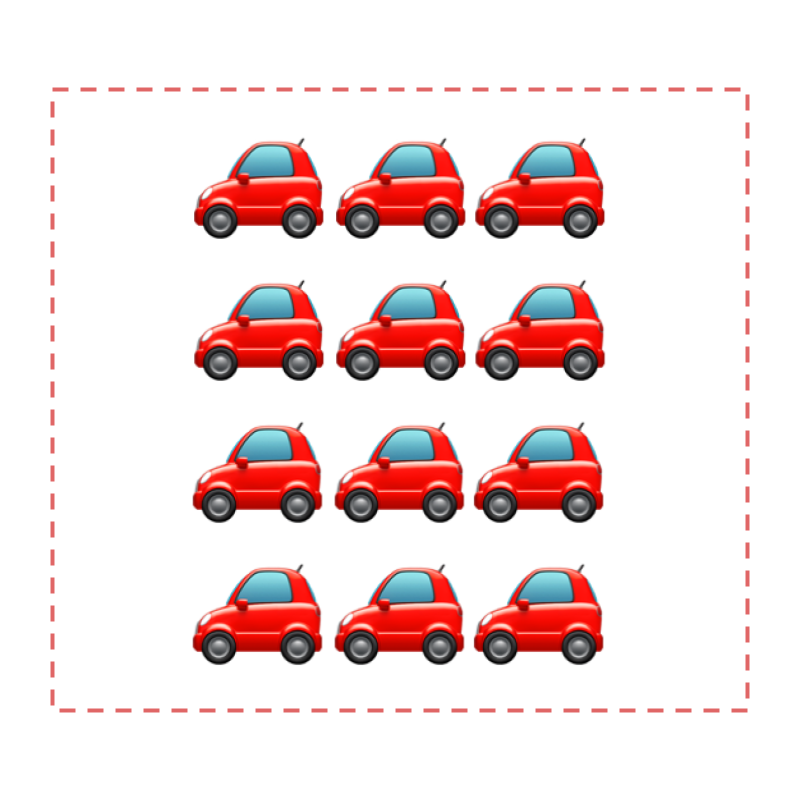There are 12$12$ cars given to 4$4$ showrooms. Each get equal number of cars.

Each showroom gets 3$3$ cars.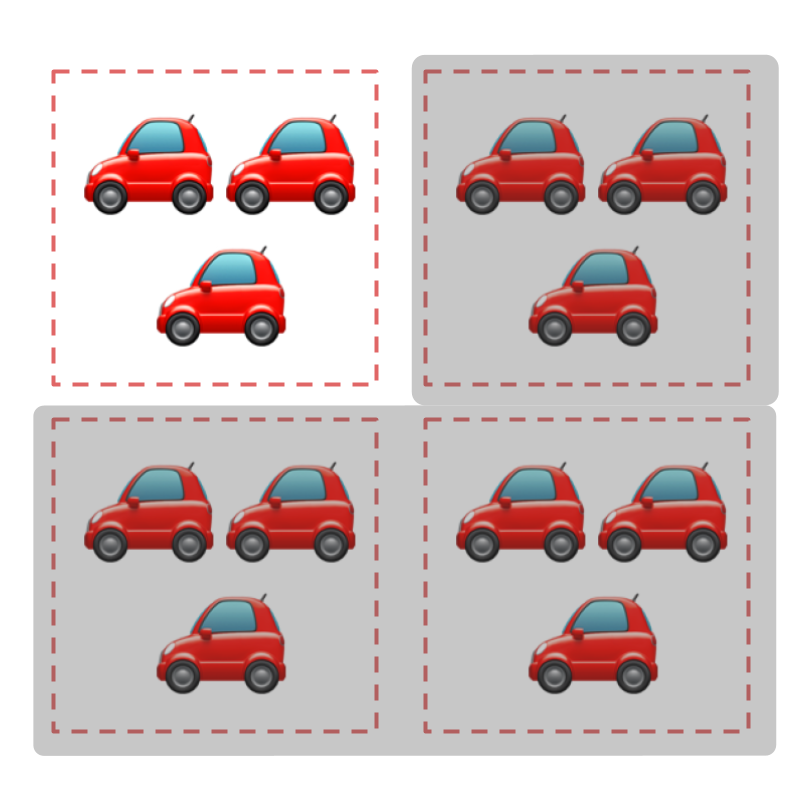Considering the figure with $12$ cars and $4$ boxes representing each of the $4$ show rooms.
$12$ is split into $4$ equal parts and one part is selected. And the counting is done from $1$ to $3$. The resulting count is $3$.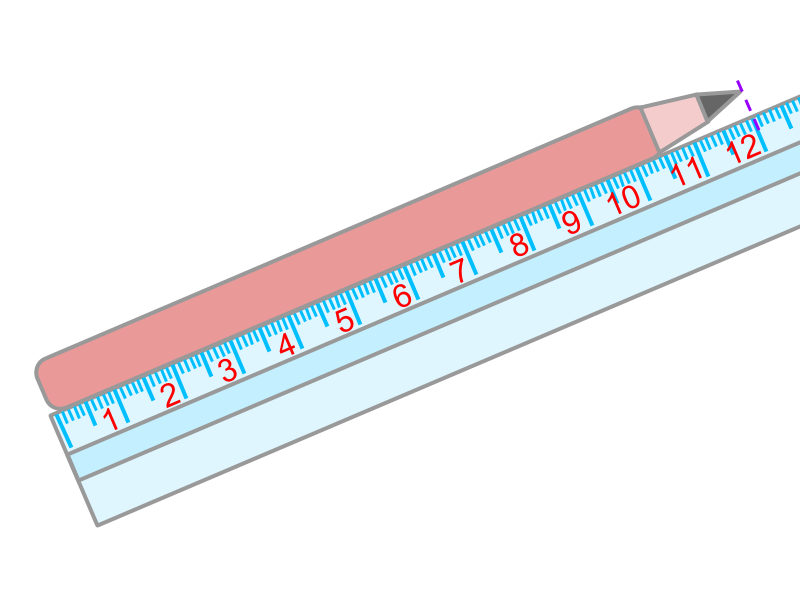A pencil is $12$ centimeters in length. If the pencil is cut into $4$ equal pieces, each piece is of length $3$ centimeters.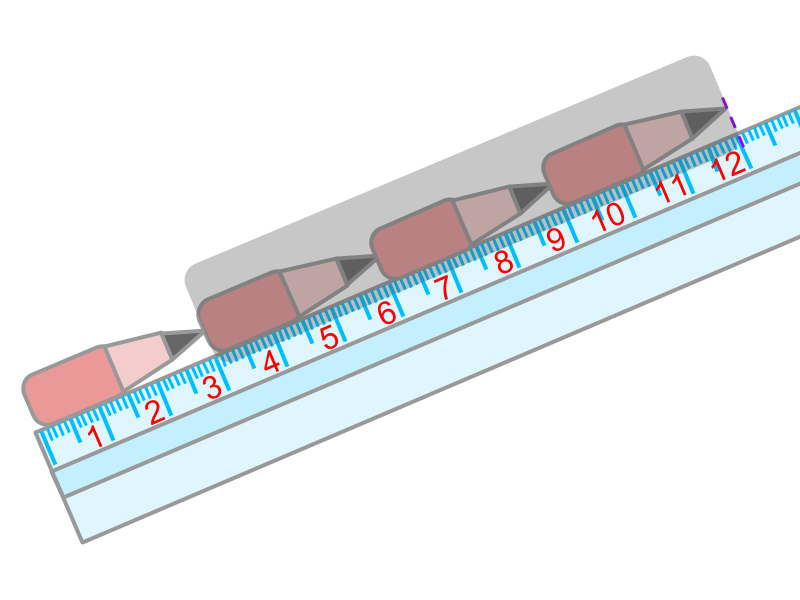A pencil is $12$ centimeters in length.
The pencil is split into $4$ equal pieces and one piece is selected. The selected piece is measured to be $3$ centimeter long.

The count or measure of quantities can be split into equal parts or divided.

The division results in the count or measure of one part of the quantity.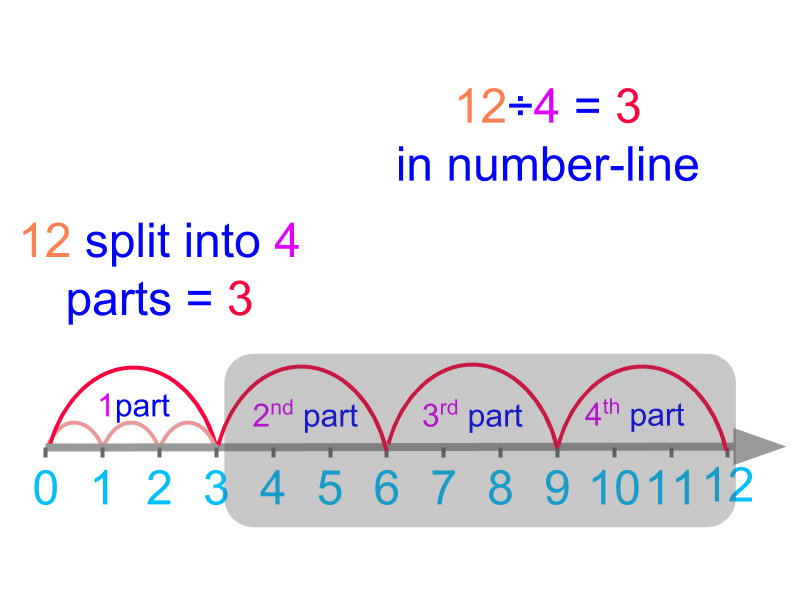Division $12 \div 4$ is given in number-line. The whole $12$ is split into $4$ equal parts. One part is chosen. The rest are grayed in the figure. The chosen part is counted in the number-line to be $3$.

dividend, divisor, quotient, remainder

The word "division" means: action of separating or splitting something.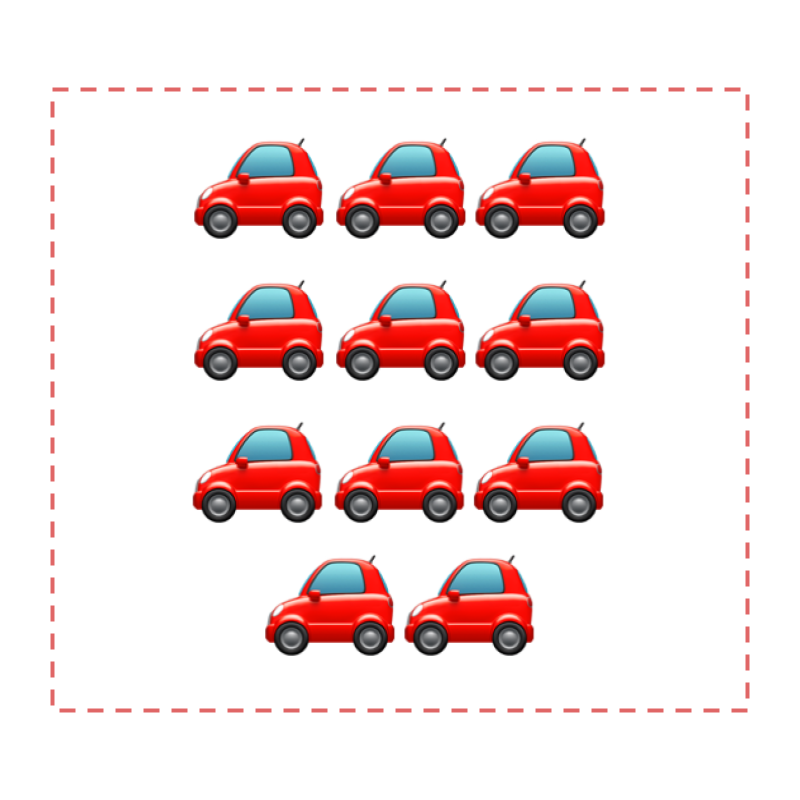There are $11$ cars given equally among $4$ showrooms. Each showroom gets $2$, and $3$ cars cannot be distributed.Considering the figure with $11$ cars and $4$ dotted-boxes representing each of the $4$ show rooms.$11$ is split into $4$ equal parts with each assigned $2$ cars. There are $3$ cars remaining. The remaining cars are not enough to assign equally to each dotted-box.

After distributing, one part is counted as $2$ and remaining amount is $3$.Division $11 \div 4$ is given in number-line. The whole $11$ is split into $4$ equal parts with $3$ remaining.

One part is chosen. The rest are grayed in the figure. The chosen part is counted in the number-line to be $2$.

Division - First Principles : Two numbers are considered, each of which represents a count or measurement. One of the amount is split into a number of parts given by the second. The amount of one part is the result.

eg: $11 \div 4 = 2$ count and $3$ remaining

$11$ is the dividend

$4$ is the divisor

$2$ is the quotient

$3$ is the remainder

The word "dividend" means: a number that is being divided.

The word "divisor" means: a number that divides another number.

The word "quotient" means: the result of a division.

The word "remainder" means: the number left over in division.

summary

Division - First Principles : Two numbers are considered, each of which represents a count or measurement. One of the amount is split into a number of parts given by the second. The amount of one part is the result.

eg: $11 \div 4 = 2$ count and $3$ remaining

$11$ is the dividend

$4$ is the divisor

$2$ is the quotient

$3$ is the remainder

$11 \div 4 = 2 \textrm{q u o t i e n t} \textrm{\mathmr{and}} 3 \textrm{r e m a \in \mathrm{de} r}$

Outline